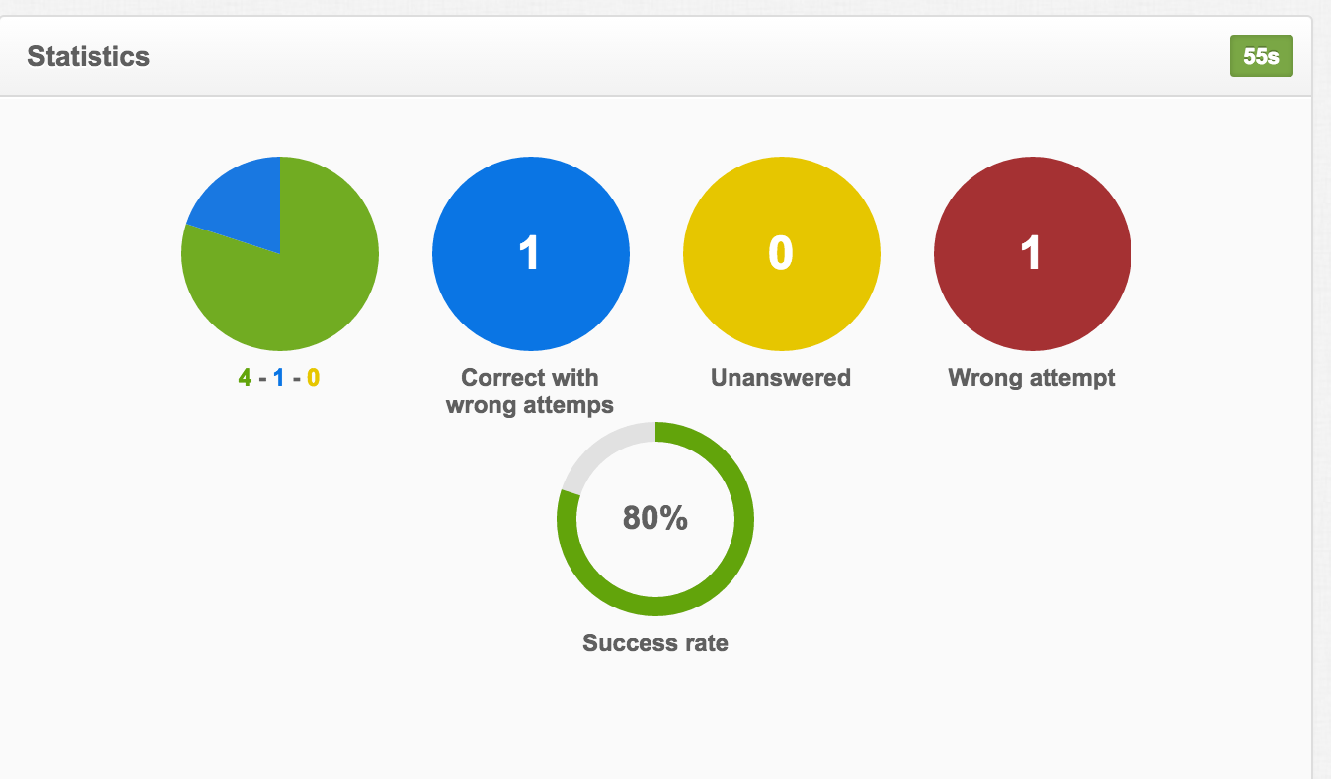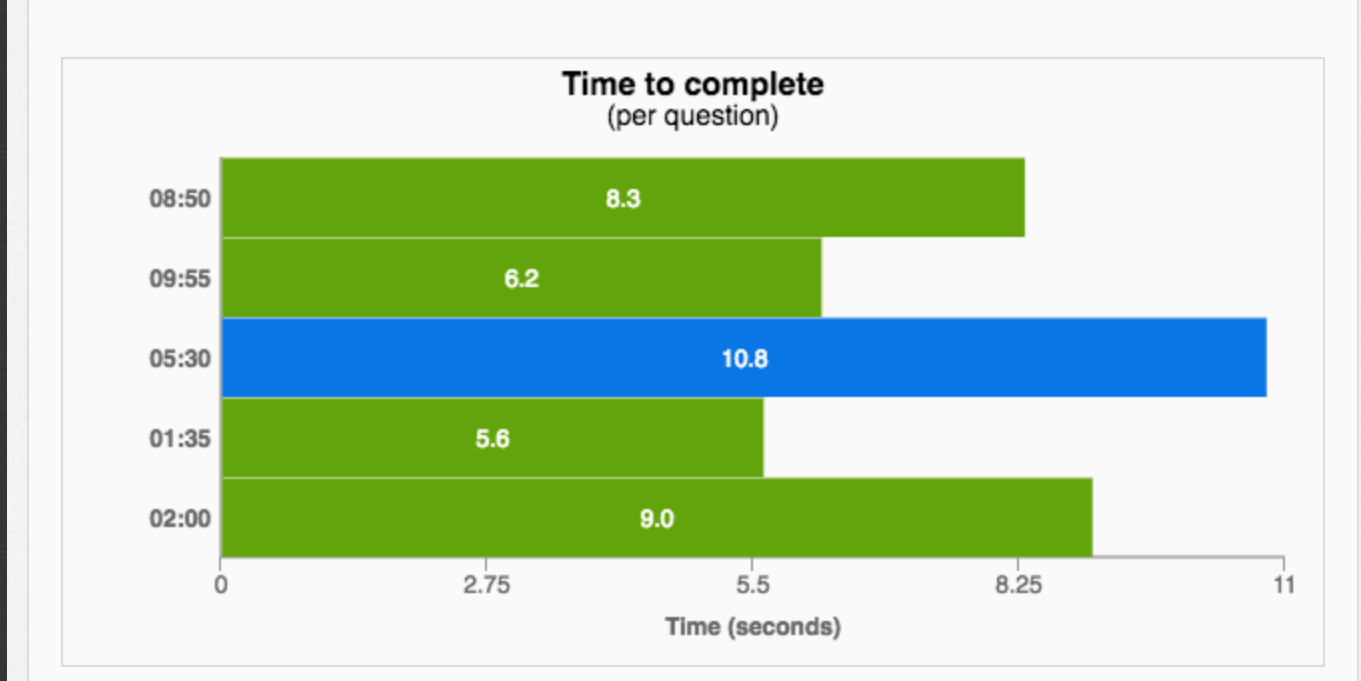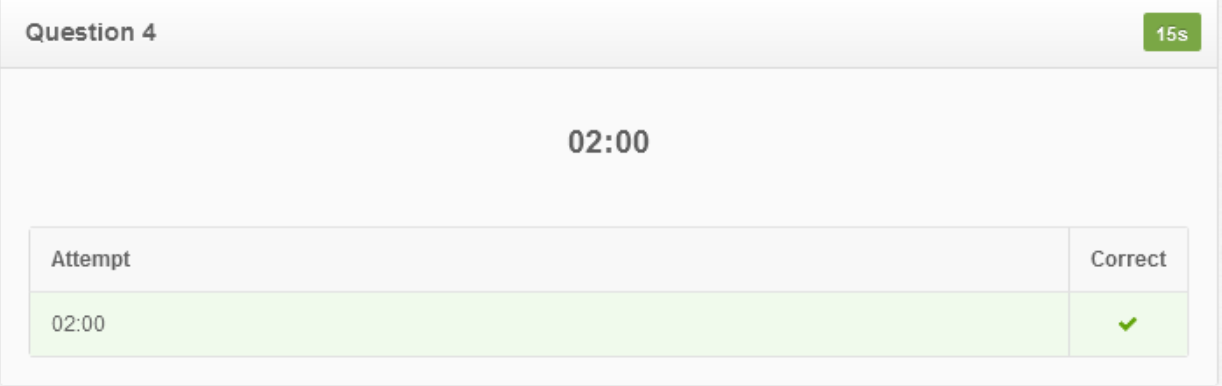### Clockoo

The “Clockoo” game helps students practice setting time on an analog clock by moving the hands of a clock to various times. They also learn the relationship between digital and analog clocks. Students set the clock to a given time by making circular hand movements. They control the hour and/or minute hands. The teacher/therapist has control of whether the student will set the time by moving one or both hands of the clock. By choosing the analog display, the student is asked to recognize the time displayed on an analog clock which appears at the side of the screen. That is the prompt. The student must set that time at the main analog clock by picking and rotating the appropriate hand(s) of the clock. Also, the teacher/therapist can pick out the time interval (Half Hour, Quarterly, etc.) on which the student should work. The student also explores the relationship among digital and analog clocks. There is a “cuckoo” that checks if an answer is right or wrong. The teacher can also activate a timer and lives to make the game more challenging. Are you ready to set the time and hear the cuckoo congratulate you?

## Supported goals in Common CoreCaliforniaFloridaNevadaNew YorkNorth CarolinaPennsylvaniaSouth CarolinaTenesseeTexasVirginia

CCSS.MATH.CONTENT.1.MD.B.3
Math
Tell and write time in hours and half-hours using analog and digital clocks.
CCSS.MATH.CONTENT.2.MD.C.7
Math
Tell and write time from analog and digital clocks to the nearest five minutes, using a.m. and p.m.
CA.MATH.1.MD.3
Math
Tell and write time. Tell and write time in hours and half-hours using analog and digital clocks.
CA.MATH.2.MD.7
Math
Work with time and money. Tell and write time from analog and digital clocks to the nearest five minutes, using a.m. and p.m. Know relationships of time (e.g., minutes in an hour, days in a month, weeks in a year).
MAFS.1.MD.2.AP.3a
Math
Tell time in whole and half hours using a digital clock
MAFS.2.MD.3.AP.7a
Math
Tell and write time in hours and half-hours using analog and digital clocks
NVAC.MATH.2.MD.C.7
Math
Work with time and money. Tell and write time from analog and digital clocks to the nearest five minutes, using a.m. and p.m.
NVAC.MATH.1.MD.B.3
Math
Tell and write time. Tell and write time in hours and half-hours using analog and digital clocks.
NY.MATH.2.MD.7
Math
Tell and write time from analog and digital clocks to the nearest five minutes, using a.m. and p.m.
NY.MATH.1.MD.3
Math
Tell and write time in hours and half-hours using analog and digital clocks. Recognize and identify coins, their names, and their value.
NC.MATH.1.MD.3
Math
Tell and write time in hours and half-hours using analog and digital clocks.
NC.MATH.2.MD.7
Math
Tell and write time from analog and digital clocks to the nearest five minutes, using a.m. and p.m.
PA.CC.MATH.1.MD.B.3
Math
Tell and write time in hours and half-hours using analog and digital clocks.
PA.CC.MATH.2.MD.C.7
Math
Tell and write time from analog and digital clocks to the nearest five minutes, using a.m. and p.m.
SC.MATH.1.MDA.3
Math
Use analog and digital clocks to tell and record time to the hour and half hour.
SC.MATH.2.MDA.6
Math
Use analog and digital clocks to tell and record time to the nearest five-minute interval using a.m. and p.m.
No supported goals.
TEKS.MATH.2.9.G
Math
Read and write time to the nearest one-minute increment using analog and digital clocks and distinguish between a.m. and p.m.
TEKS.MATH.1.7.E
Math
Tell time to the hour and half hour using analog and digital clocks.
VASOL.MATH.K.M.9
Math
The student will tell time to the hour, using analog and digital clocks.
VASOL.MATH.2.M.12
Math
The student will tell and write time to the nearest five minutes, using analog and digital clocks.
VASOL.MATH.1.M.8
Math
The student will tell time to the half-hour, using analog and digital clocks.

## Settings

### I CAN statement

Music

Disable/enable background music

• On
• Off
Sound effects

Disable/enable sound effects while selecting an item.

• On
• Off

I CAN understand that there are sounds when I choose an item.

Select hand
• Left
• Right

I CAN move the big hand of the clock with my left hand.

Time to select item

·Time to remain stable to select an item

·Reduce the time if the child shows arm stability

• 0.5 sec
• 1 sec
• 1.5 sec
• 2 secs
• 2.5 secs
• 3 secs

I CAN hold my left hand still for 3 seconds to select an item.

Timer

Time to finish selecting the side

Disable this is if the student feels pressure

• On
• Off

I CAN answer 5 questions in five minutes.

Timer value
• 10 secs
• 20 secs
• 30 secs
• 40 secs
Game lives

Game lives are either in the shape of pink clouds or cream flowers surrounding the clock. A life will be pulled off the screen when the answer is wrong. There are 3 lives.

• On
• Off

I CAN understand my mistakes when the game lives decrease.

Game type

This is the clock that the student has to match the time with which will be shown on the right side of the screen. It can be set as analog or digital clocks.

• Analog to analog
• Digital to analog
• Elapsed/Extended time - Analog
• Elapsed/Extended time - Digital

I CAN read time from the digital clock.

Number of questions

The amount of questions the student has to answer.

• 5 questions
• 10 questions
• 15 questions
• 20 questions

Time difference
• 5 min
• 15 min
• Half hour
• Hour
Minute hand step

This function determines how complicated the clock should be. The hour step is set if the student should adjust only the hour (O’clock), half an hour is such as 1:30, 15 min. can be 1:15 or 1:45, and 5 min. can be 1:05 or 2:20. The minute hand will make the game more challenging for the students.

• 5 min
• 15 min
• Half hour
• Hour

I CAN set O’clock time.

I CAN set a time that has minutes.

Interact with

This function determines which hand the student has to work with in order to set the clock. It can be the hour hand only, the minute hand only, or both.

• Hour hand
• Minute hand
• Hour and minute hand

I CAN adjust the hour hand to make 4:00.

I CAN adjust the minute hand to make 1:30.

Realistic hour hand

This setting determines the realistic function like a real clock.

• On
• Off

I CAN adjust the hour & the minute hand to set the time in a clock that reacts like a real clock.

## ReportsPie chart data

·      This shows statistics of how many times the student gave correct, wrong or no answers and how many times the student tried to find the correct answers

The Time is shown on the top right corner. The teacher can use the time to tell whether the task is challenging enough for the students.Table data

·      This shows the time a student takes to complete a question. The student spent the longest time to complete 05:30 (10.8 s).

This data can reveal certain patterns that the students are struggling with.·      This shows the time per question in details. The time period is shown on the right and the task that the student completed is also displayed. In this case, the student worked on 02:00 and got it correct in 15s.